# Axis 6: Optimization algorithms: deterministic and stochastic methods

In information geometry, statistical data take values ​​in sets equipped with a Riemannian structure, with finite or infinite dimension. The estimators of quantities relating to these data are averages, medians, or more generally p-averages of these data. Stochastic algorithms to find these p-averages are very useful for all of the practical applications that have been developed in the publications listed below. It may be mentioned in particular applications in processing stationary radar signals. A very important issue is to consider non-stationary signals. To this end, we need to work on paths spaces in Riemannian manifolds, and develop a good notion of metric, distance, and average for these paths.

New stochastic algorithms of Robbins-Monro type have also been proposed to efficiently estimate the unknown parameters of deformation models. These estimation procedures are implemented on real ECG data to detect cardiac arrhythmia problems.

Proximal methods have been extremely successful in image processing to provide efficient algorithms to compute the solutions of the considered problems. A major theme of the team is the study of the convergence of such algorithms, their speed, and robustness to errors.

# Non parametric noise estimation

In order to provide a fully automatic denoising algorithm, we have developed an automatic noise estimation method that relies on the non-parametric detection of homogeneous areas. First, the homogeneous regions of the image are detected by computing Kendall’s rank correlation coefficient . Computed on neighboring pixel sequences, it indicates the dependancy between neighbors, hence reflects the presence of structure inside an image block. This test is non-parametric, so the performance of the detection is independant of the noise statistical distribution. Once the homogeneous areas are detected, the noise level function, i.e., the function of the noise variance with respect to the image intensities, is estimated as a second order polynomial minimizing the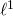error on the statistics of these regions.

Matlab implementation of the noise estimation algorithm

Related papers:

– C. Sutour, C.-A. Deledalle et J.-F. Aujol. Estimation of the noise level function based on a non-parametric detection of homogeneous image regions. Submitted to Siam Journal on Imaging Sciences, 2015.

– C. Sutour, C.-A. Deledalle et J.-F. Aujol. Estimation du niveau de bruit par la détection non paramétrique de zones homogènes. Submitted to Gretsi, 2015.

## References

 Buades, A., Coll, B., and Morel, J.-M. (2005). A review of image denoising algorithms, with a new one. Multiscale Modeling and Simulation, 4(2): 490–530.

The denoising algorithm that has been developed is based on an adaptive regularization of the NL-means . The proposed model is the following:

(1)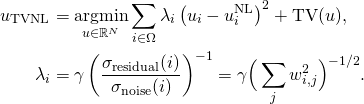where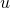is the solution obtained with the NL-means algorithm, TV refers to the total variation of the image and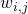is the weight that measures the similarity between the patch of indexand the patch of indexin the NL-means algorithm. The ratioreflects the noise variance reduction performed by the NL-means. This formulation allows locally adaptive regularization of the NL-means solution, thanks to a confidence index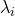that reflects the quality of the denoising performed by the NL-means.

This model can be adapted to the different noise statistics belonging to the exponential family (Gaussian, Poisson, multiplicative…). It can also be adapted to video denoising thanks to the use of 3D patches combined to a spatio-temporal TV regularization.

Matlab implementation of RNL

Results of video denoising with R-NL and comparisons

Related papers:
1. C. Sutour, C.-A. Deledalle et J.-F. Aujol. Adaptive regularization of the NL-means : Application to image and video denoising. IEEE Transactions on image processing, vol. 23(8) : 3506-3521, 2014.

2. C. Sutour, J.-F. Aujol, C.-A. Deledalle et J.-P. Domenger. Adaptive regularization of the NL-means for video denoising. International Conference on Image Processing (ICIP), pages 2704–2708. IEEE, 2014.

3. C. Sutour, J.-F. Aujol et C.-A. Deledalle. TV-NL : Une coopération entre les NL-means et les méthodes variationnelles. Gretsi, 2013.

## References

 Buades, A., Coll, B., and Morel, J.-M. (2005). A review of image denoising algorithms, with a new one. Multiscale Modeling and Simulation, 4(2): 490–530.

# Image colorization

Colorization is an important issue for example for the restoration of old documents, but also for the entertainment industry. The methods fall into two categories, manual methods and example-based methods for which the user provides a color image which serves as a source of information. The comparison of textures used to extract color. Regularization is then required, that is carried out in our case by minimizing a non-convex function. To propose a reasonable model for colorization and an efficient algorithm to minimize the proposed functional constituted the bulk of the beginning of the thesis.

However, methods-based example exhibited difficulty in finding a relevant source image. It is difficult to find a relevant source image for a given colorization, and the method fails frequently, just for example, when a color is not present in the source image, or when two smooth portions or similar textures expressed a different color. To avoid these issues, we proposed a method called collaborative colorization, based on an interactive method in which the user supervises the result. It has the ability to integrate color points in the result where the result appears unsatisfactory. This new information is integrated into the process by exploiting the non-convexity of the model.# Stein Unbiased GrAdient estimator of the Risk

Algorithms to solve variational regularization of ill-posed inverse problems usually involve operators that depend on a collection of continuous parameters. When these operators enjoy some (local) regularity, these parameters can be selected using the so-called Stein Unbiased Risk Estimate (SURE). While this selection is usually performed by exhaustive search, we address in this work the problem of using the SURE to efficiently optimize for a collection of continuous parameters of the model. When considering non-smooth regularizers, such as the popular l1-norm corresponding to soft-thresholding mapping, the SURE is a discontinuous function of the parameters preventing the use of gradient descent optimization techniques. Instead, we focus on an approximation of the SURE based on finite differences as proposed in (Ramani et al., 2008). Under mild assumptions on the estimation mapping, we show that this approximation is a weakly differentiable function of the parameters and its weak gradient, coined the Stein Unbiased GrAdient estimator of the Risk (SUGAR), provides an asymptotically (with respect to the data dimension) unbiased estimate of the gradient of the risk. Moreover, in the particular case of soft-thresholding, the SUGAR is proved to be also a consistent estimator. The SUGAR can then be used as a basis to perform a quasi-Newton optimization. The computation of the SUGAR relies on the closed-form (weak) differentiation of the non-smooth function. We provide its expression for a large class of iterative proximal splitting methods and apply our strategy to regularizations involving non-smooth convex structured penalties. Illustrations on various image restoration and matrix completion problems are given.

# Associated publications and source codes:

Charles-Alban Deledalle, Samuel Vaiter, Gabriel Peyré and Jalal Fadili
Stein Unbiased GrAdient estimator of the Risk (SUGAR) for multiple parameter selection,
Technical report HAL, hal-00987295 (HAL)

MATLAB source codes available from GitHub.

# Edge based multi-modal registration

In order to perform multi-modal fusion between images obtained from a light intensifier (LI) and IR (infra-red) images, hence providing multi-sources information to the pilot, it is necessary to perform registration between the two modalities. They reflect different properties of the scene, so the selected approach is based on the geometric information and the edges of each image.

The goal is to find the transformationthat transfers the IL image into the frame of reference of the IR image by minimizing the following energy:

(1)This metric is based on the edges of each image, and it only takes into account the edges that occur in both modalitites, which makes it insensitive to outliers.
Thanks to a gradient descent based optimization scheme, it is possible to quickly evaluate to optimal transformation between the two modalities.

Registration results on real data

Related papers:
C. Sutour, J.-F. Aujol, C.-A. Deledalle et B.D. De-Senneville. Edge-based multi-modal registration and application for night vision devices. Journal of Mathematical Imaging and Vision, pages 1–20, 2015.

# NL-SAR: Non-Local framework for (Pol)(In)SAR denoising

NL-SAR (2013-2014)
Open-source software distributed under CeCILL license to perform adaptive non-local (Pol)(In)SAR filtering. Interface in command line, IDL, Matlab, Python and C dynamic library.